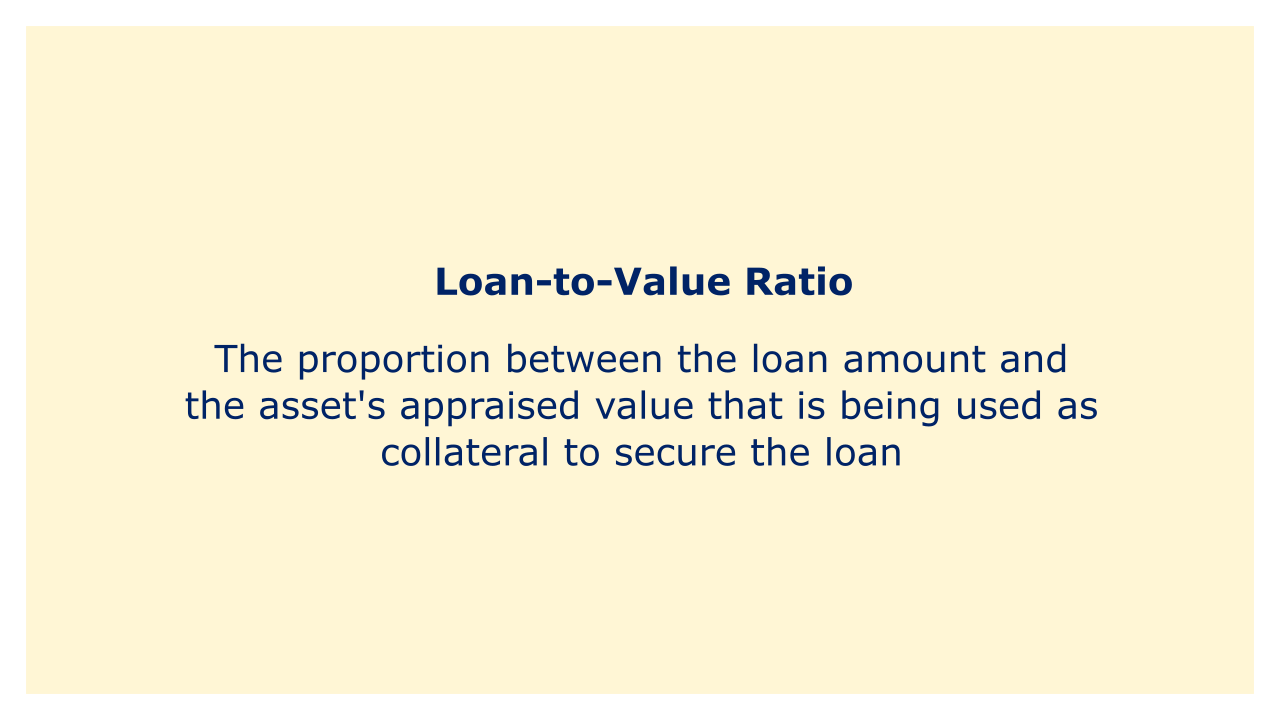# Loan-to-Value RatioImage: Moneybestpal.com

### Lenders use the loan-to-value (LTV) ratio as a financial statistic to assess the risk of a loan. It is described as the proportion between the loan amount and the asset's appraised value that is being used as collateral to secure the loan. The LTV ratio is a critical component in determining whether a lender will approve a loan application and the terms of the loan. It is stated as a percentage.

The LTV ratio is a numerical measurement of how much a borrower is borrowing in relation to the value of the asset pledged as security. If a borrower wishes to buy a house valued at \$500,000 and the lender approves a loan for \$400,000, for instance, the LTV ratio would be computed as 400,000/500,000 = 0.8 or 80%.

Due to its ability to quantify the equity that the borrower has in the collateral, the LTV ratio is used by lenders to evaluate the risk of a loan. Due to the less amount of equity in the asset available to cover the loan in the case of failure, a higher LTV ratio puts the lender at a higher risk. Because of this, lenders often have a maximum LTV ratio they would accept for a specific type of loan.

Lenders often want a maximum LTV ratio of 80% in mortgage loans, for instance. For a borrower to be approved for a loan, a down payment of at least 20% of the value of the home is required. The lender may impose private mortgage insurance (PMI) as a safeguard against the higher risk if the borrower is unable to put down a 20% deposit.
Tags